1. /
2. CBSE
3. /
4. Class 07
5. /
6. Mathematics
7. /
8. NCERT Solutions for Class...

# NCERT Solutions for Class 8 Maths Exercise 7.2### myCBSEguide App

Download the app to get CBSE Sample Papers 2023-24, NCERT Solutions (Revised), Most Important Questions, Previous Year Question Bank, Mock Tests, and Detailed Notes.

NCERT solutions for Class 8 Maths Cubes and Cube Roots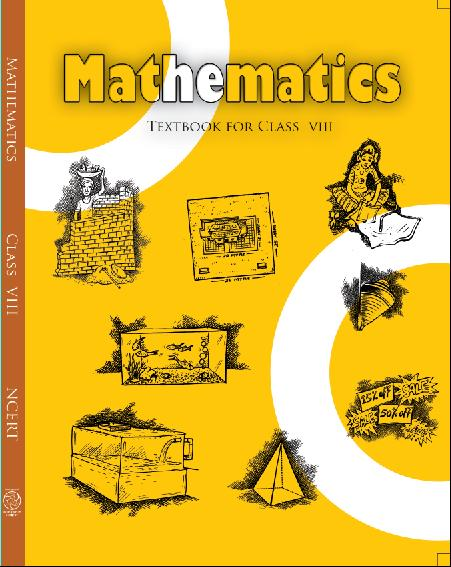## NCERT Solutions for Class 8 Maths Cubes and Cube Roots

###### 1. Find the cube root of each of the following numbers by prime factorization method:

(i) 64

(ii) 512

(iii) 10648

(iv) 27000

(v) 15625

(vi) 13824

(vii) 110592

(viii) 46656

(ix) 175616

(x) 91125

Ans. (i) 64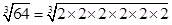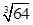=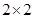= 4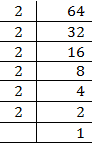(ii) 512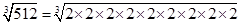=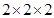= 8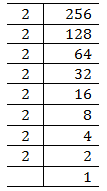(iii) 10648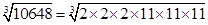=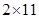= 22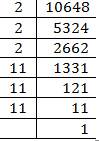###### (iv) 27000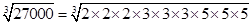=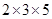= 30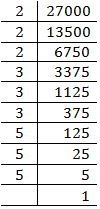###### (v) 15625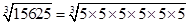=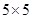= 25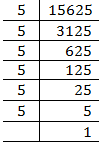(vi) 13824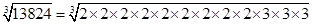=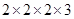= 24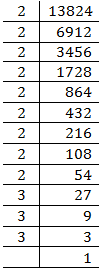(vii) 110592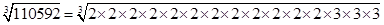=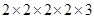= 48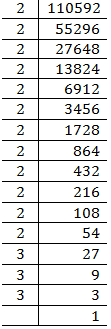(viii) 46656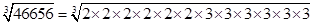=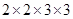= 36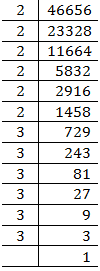(ix) 175616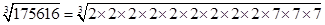=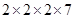= 56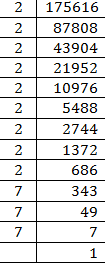(x) 91125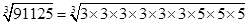=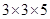= 45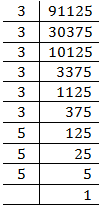NCERT Solutions for Class 8 Maths Exercise 7.2

###### 2. State true or false:

(i) Cube of any odd number is even.

(ii) A perfect cube does not end with two zeroes.

(iii) If square of a number ends with 5, then its cube ends with 25.

(iv) There is no perfect cube which ends with 8.

(v) The cube of a two digit number may be a three digit number.

(vi) The cube of a two digit number may have seven or more digits.

(vii) The cube of a single digit number may be a single digit number.

Ans. (i) False

Since,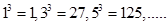are all odd.

(ii) True

Since, a perfect cube ends with three zeroes. e.g.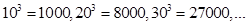so on

(iii) False

Since,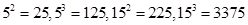(Did not end with 25)

(iv) False

Since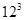= 1728

[Ends with 8]

And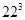= 10648

[Ends with 8]

(v) False Since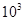= 1000

[Four digit number]

And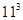= 1331

[Four digit number]

(vi) False Since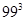= 970299

[Six digit number]

(vii) True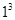= 1

[Single digit number]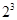= 8

[Single digit number]

NCERT Solutions for Class 8 Maths Exercise 7.2

###### 3. You are told that 1,331 is a perfect cube. Can you guess with factorization what is its cube root? Similarly guess the cube roots of 4913, 12167, 32768.

Ans. We know that= 1000 and Possible cube of= 1331

Since, cube of unit’s digit= 1

Therefore, cube root of 1331 is 11.

4913

We know that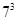= 343

Next number comes with 7 as unit place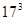= 4913

Hence, cube root of 4913 is 17.

12167

We know that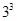= 27

Here in cube, ones digit is 7

Now next number with 3 as ones digit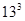= 2197

And next number with 3 as ones digit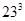= 12167

Hence cube root of 12167 is 23.

32768

We know that= 8

Here in cube, ones digit is 8

Now next number with 2 as ones digit= 1728

And next number with 2 as ones digit= 10648

And next number with 2 as ones digit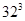= 32768

Hence cube root of 32768 is 32.

## NCERT Solutions for Class 8 Maths Exercise 7.2

NCERT Solutions Class 8 Mathematics PDF (Download) Free from myCBSEguide app and myCBSEguide website. Ncert solution class 8 Mathematics includes text book solutions from Class 8 Maths Book . NCERT Solutions for CBSE Class 8 Maths have total 16 chapters. 8 Maths NCERT Solutions in PDF for free Download on our website. Ncert class 8 solutions PDF and Maths ncert class 8 PDF solutions with latest modifications and as per the latest CBSE syllabus are only available in myCBSEguide.

## CBSE app for Class 8

To download NCERT Solutions for class 8 Social Science, Computer Science, Home Science,Hindi ,English, Maths Science do check myCBSEguide app or website. myCBSEguide provides sample papers with solution, test papers for chapter-wise practice, NCERT solutions, NCERT Exemplar solutions, quick revision notes for ready reference, CBSE guess papers and CBSE important question papers. Sample Paper all are made available through the best app for CBSE students and myCBSEguide website.Test Generator

Create question paper PDF and online tests with your own name & logo in minutes.myCBSEguide

Question Bank, Mock Tests, Exam Papers, NCERT Solutions, Sample Papers, Notes

### 10 thoughts on “NCERT Solutions for Class 8 Maths Exercise 7.2”

1. We can learn many things from it

2. Good

3. thanks